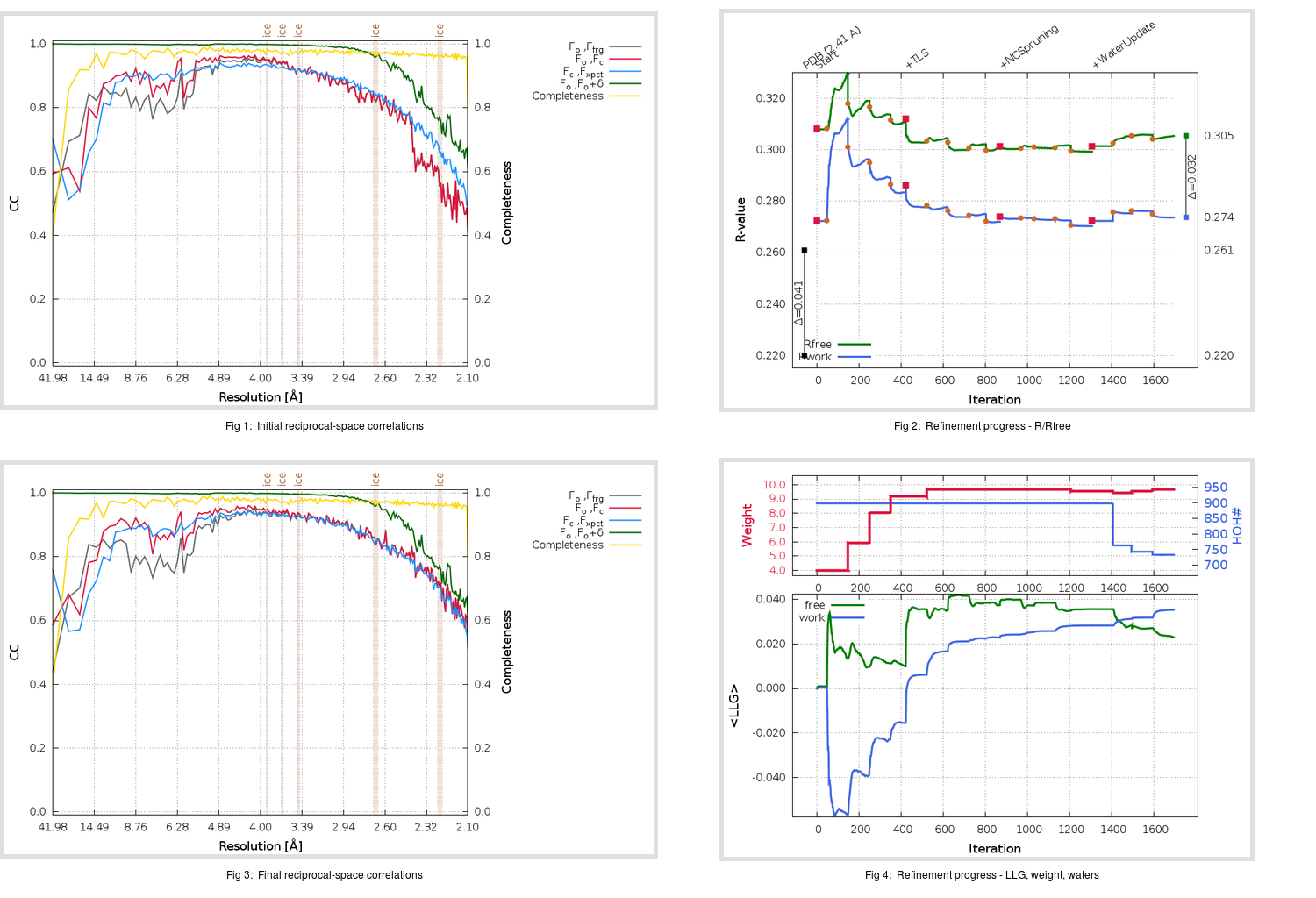Content:

```    Diffraction limits & principal axes of ellipsoid fitted to diffraction cut-off surface:
2.841         0.6219   0.6901   0.3701       0.332 a* + 0.432 b* + 0.838 c*
2.655        -0.6343   0.7211  -0.2788      -0.513 a* + 0.457 b* - 0.727 c*
2.565        -0.4592  -0.0613   0.8862      -0.172 a* - 0.060 b* + 0.983 c*
```

## Deposited

` `
 Date deposited Date data collection Resolution R, Rfree 20100825 20091208 2.41 0.2170 0.2610

Molprobity (CCP4 7.0 version) summary:

```Ramachandran outliers =   0.22 %
favored =  97.00 %
Rotamer outliers      =   4.84 %
C-beta deviations     =     0
Clashscore            =   9.57
RMS(bonds)            =   0.0089
RMS(angles)           =   1.08
MolProbity score      =   2.20
Resolution            =   2.41
R-work                =   0.2170
R-free                =   0.2610
```

```Number of waters      =   898

<B> (all atoms) =   65.50 ( sd =   24.93 ) for      18860 non-hydrogen atoms
<B>   (protein) =   62.40 ( sd =   20.74 ) for      14788 non-hydrogen atoms
<B>     (water) =   64.99 ( sd =   13.44 ) for        898 non-hydrogen atoms
<B>    (others) =   80.27 ( sd =   37.44 ) for       3174 non-hydrogen atoms

B min/max       (all non-hydrogen atoms) =   28.08 /  196.93
B min/max   (protein non-hydrogen atoms) =   28.08 /  163.37
B min/max     (water non-hydrogen atoms) =   30.20 /  144.57
B min/max     (other non-hydrogen atoms) =   31.14 /  196.93
```

## BUSTER (re-)refinement

` `

Molprobity (CCP4 7.0 version) summary: Additional analysis:

```Number of waters      =   733
```

Refinement progression:Results:

` `
 File Remark 3OLB_aB_refine.01_04_refine.pdb.gz exact refinement commands are in header 3OLB_aB_refine.01_04_refine.mtz.gz including original deposited data and several re-refinement map coefficients 3OLB_aB_refine.01_04_BUSTER_model.cif.gz including any non-standard compound restraints 3OLB_aB_refine.01_04_BUSTER_refln.cif.gz